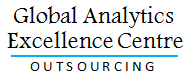# White Papers

### Techniques in DNA Data Mining

The main concern of data mining is analysis of data. Its main objective is to detect patterns automatically in any data set through minimum user input and efforts. There is a vast set of data mining tools and techniques which can be applied in varied fields or myriad forms. It can also be employed for making decision and for forecasting future trends of a market.
Techniques in DNA Data Mining

### SAS versus R

There is a big argument going on the pros and cons of SAS and R technologies for statistical computing now-a-days. Entrepreneurs are in search of the right answer to find which one is more cost effective for statistical modeling. They want to know, “Will R be able to replace SAS in near future or not?” Here is a brief contrast between SAS and R which will help you to understand the importance and worth of these two software languages.
SAS versus R

### Regression Analysis in Data Analytic

In the domain of statistics, the most commonly used statistical technique is Regression Analysis which is used to estimate particular relationships among variables. Under this technique, the main focus is upon the relationship between dependent variable and any one or more independent variables. There are several techniques within this analysis that are used for modeling and analyzing several variables.
Regression Analysis in Data Analytic

### Linear Regression

Linear regression is one of the prime statistical tools used to study or analyze the relationship between two variables, X and Y. The purpose of this widely used tool is to analyze the correlation of a response variable to the notified explanatory variable. This statistical method is also employed to forecast future values from past values.
Linear Regression

### Logistic Regression

Logistic regression is a statistical model which is used for predicting the possibility of existence of an event. Generally, this model makes use of various predictor variables which may be either numerical or divisions.
Logistic Regression

### Heat Maps: The new Age of Data Visualization

A Heat map is a unique two-dimensional display of data in which values are dictated in the form of colours. Generally there are two aspects of heat map, fractal maps and tree maps, and both of them employ similar colour coding to represent individual variables. The more elaborate heat maps display complex data sets in perceivable and simplified terms.
Heat Maps: The new Age of Data Visualization## Testimonial

"Started with one assignment, they satisfy all my analytics needs. Good quality, cost effective - Our godsend analysis partner we much needed."

Director, E-commerce company, UK